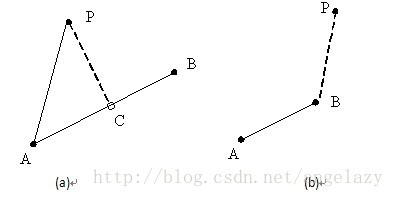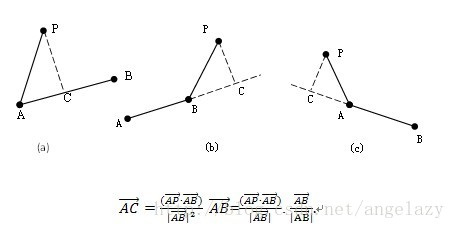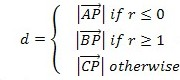点到线段的最短距离1 （a）最短距离为点P与其在线段AB上投影C之间的线段PC

（b）最短距离为点P与端点B（或A）所构成的线段PB（或PA）

3、方法三——矢量算法(B)所示的情况，那么r ≥1;
(C)所示的情况，那么得到r ≤0;double PointToSegDist(double x, double y, double x1, double y1, double x2, double y2)
{
double cross = (x2 - x1) * (x - x1) + (y2 - y1) * (y - y1);
if (cross <= 0) return Math.Sqrt((x - x1) * (x - x1) + (y - y1) * (y - y1));

double d2 = (x2 - x1) * (x2 - x1) + (y2 - y1) * (y2 - y1);
if (cross >= d2) return Math.Sqrt((x - x2) * (x - x2) + (y - y2) * (y - y2));

double r = cross / d2;
double px = x1 + (x2 - x1) * r;
double py = y1 + (y2 - y1) * r;
return Math.Sqrt((x - px) * (x - px) + (py - y) * (py - y));
}

posted @ 2018-07-24 13:51  采男孩的小蘑菇  阅读(10922)  评论(2编辑  收藏  举报